Four, Five or Six Band Resistor Color Code Calculator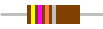CALCULATOR   INSTRUCTIONS

This calculator solves for 4, 5 or 6 band resistors and is quite simple to use. To calculate a four band resistor value, use the middle four "drop" boxes then click on the "Calc 4 Band" button. For a five or six band resistor, you can use all six boxes but all 6 do not necessarily have to be used - the "Temperature Coefficient" box, for example. After you have selected the 6 "drop box" choices, remember to click the "Calc 5 Band" button for your answer.
If you have calculated a 5 or 6 band resistor, and go back to calculating a 4 band resistor, the two drop boxes on the ends (far left and far right) will not clear but this is perfectly all right. When calculating 4 band resistors, the values of the drop boxes on the ends do not enter into the calculations in any manner.

 Four   Band   Resistors   Resistors are electronic components that oppose the flow of electricity and the resistance is measured in ohms. For larger values, kilohms (1,000 ohms) and megohms (1,000,000 ohms) are used. For example 3,300 ohms equals 3.3 kilohms or just 3.3 k and 1,500,000 ohms equals 1.5 megohms or 1.5 meg. Color "bands" are used to indicate the resistance value with each color signifying a number and these color bands are grouped closer to one end of the resistor than the other.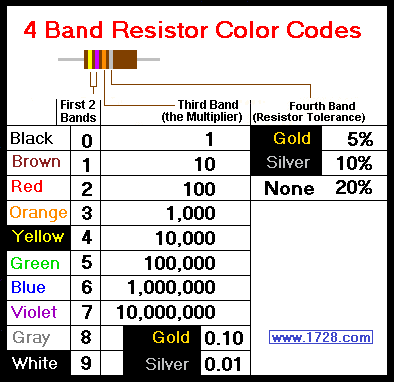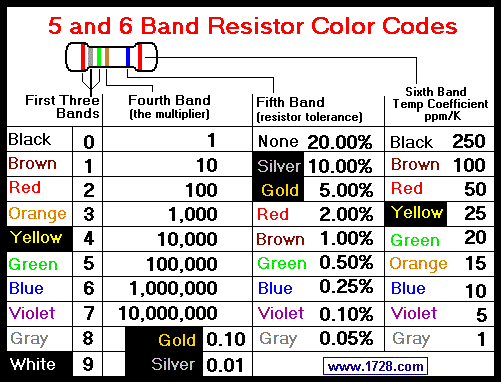As can be seen in the above 4 Band Resistor Color Codes chart, the first two color bands have values of brown = 1, red = 2, orange = 3 and so on.

The third color band is the multiplier of the first 2 bands. Here, black is 1, brown is 10, red is 100 and so on. Putting this in other words, the value of the third band (the multiplier) is the number 10 raised to the power of the color code. For example, red in the third band is 10² or 100.
This third band also has 2 new colors where gold = .1 and silver = .01.

The 4th band is the resistor's tolerance and shows how precisely the resistor was manufactured. Gold = 5%, silver = 10% and no band whatsoever = 20%.

Now that we know the values of each color, let's try calculating a few examples of resistance values.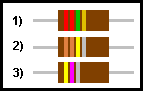Looking at resistor #1, we see the colors red red green gold.
The Color Codes chart "translates" this into 2 2 and 100,000
which equals 2 2 ×100,000 or 2,200,000 ohms and don't forget the gold 4th band which indicates a 5% tolerance.

Resistor #2 has the colors orange orange yellow silver which "translates" into 3 3 ×10,000 or 330,000 ohms and a tolerance of 10%.

Resistor #3 has the colors yellow violet silver meaning 4 7 ×.01 or .47 ohms and no fourth band indicates a 20% tolerance.

Five Band Resistors

Use the 5 Band Chart to solve these next problems.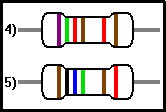For resistor 4, we see the first 3 bands are violet, green and red which "translate" into 7, 5 and 2. Looking at the fourth band (the multiplier), we see it is brown and has a value of 10.
So, the resistance value is 7 5 2 × 10 which equals 7,520 ohms or 7.52 K ohms.
Band 5 is red which indicates a 2 per cent tolerance and a brown sixth band means that the temperature coefficient is 100 parts per million (ppm).

Examining resistor 5, the first 3 bands are brown, black and blue and the fourth band (the multiplier) is green. So, these colors convert into 1 0 6 × 100,000 which calculates to 10,600,000 ohms or 10.6 Meg ohms.
The brown 5th band and the red 6th band mean that the resistor has a 1% tolerance and a 50 ppm temperature coefficient.

If you've read these instructions, you probably have a good understanding of determining a resistor's value from its colors. Then again, there's always the calculator which makes things much easier to solve.

A related resistor calculator can be found here:
Resistors In Parallel

_____________________